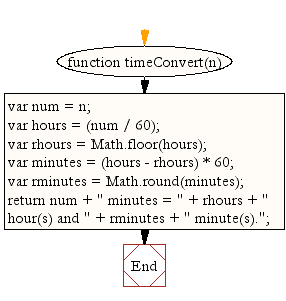# JavaScript: Return the number of minutes in hours and minutes

## JavaScript Datetime: Exercise-13 with Solution

Write a JavaScript function that will return the number of minutes in hours and minutes.

Test Data :
console.log(timeConvert(200));
Output :
"200 minutes = 3 hour(s) and 20 minute(s)."

Sample Solution:-

HTML Code:

``````<!DOCTYPE html>
<html>
<head>
<meta charset="utf-8">
<title>Return the number of minutes in hours and minutes.</title>
</head>
<body>

</body>
</html>
```
```

JavaScript Code:

``````function timeConvert(n) {
var num = n;
var hours = (num / 60);
var rhours = Math.floor(hours);
var minutes = (hours - rhours) * 60;
var rminutes = Math.round(minutes);
return num + " minutes = " + rhours + " hour(s) and " + rminutes + " minute(s).";
}

console.log(timeConvert(200));
```
```

Sample Output:

```200 minutes = 3 hour(s) and 20 minute(s).
```

Flowchart:Live Demo:

See the Pen JavaScript - Return the number of minutes in hours and minutes-date-ex- 13 by w3resource (@w3resource) on CodePen.

Improve this sample solution and post your code through Disqus

What is the difficulty level of this exercise?

﻿

## JavaScript: Tips of the Day

Convert tabs to spaces, where each tab corresponds to count spaces

Example:

```const tips_expandTabs = (str, count) => str.replace(/\t/g, ' '.repeat(count));
console.log(tips_expandTabs('\t\tw3resource', 4));
```

Output:

```"        w3resource"
```